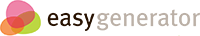# Basic Music Notation

## Treble Staff

### Question 1

• A
• B
• E
• G

Observe this diagram of a treble staff.Which of the following notes is shown above?

### Question 2

• B
• C
• F
• G

Observe this diagram of a treble staff.Which of the following notes is shown above?

### Question 3

• A A F C
• E G E C
• B F F A
• C B D C

Observe this diagram of a treble staff.Which of the following note sequences is shown above?

### Question 4

• A D F E
• B C G D
• D F E B
• C A G C

Observe this diagram of a treble staff.Which of the following note sequences is shown above?

### Question 5

Observe this diagram of a treble staff.Using the numbers on the diagram, match each number with the corresponding note name. Place one letter (A, B, C, D, E, F, or G) in each blank. Every letter A–G will be used at least once. Some letters appear twice.

1

2

3

4

5

6

7

8

9

## Notes

### Question 1

• Eighth note.
• Quarter note.
• Half note.
• Whole note.

Consider the following symbol:Which note is pictured above?

### Question 2

• Eighth note.
• Quarter note.
• Half note.
• Whole note.

Consider the following symbol:Which note is pictured above?

### Question 3

••••Select the quarter note:

### Question 4

••••Of the following symbols, which indicates the longest note?

### Question 5

••••The following symbols have been placed in random order.Select the choice below that orders these notes from SHORTEST in duration to LONGEST in duration.

## Rests

### Question 1

• Eighth rest.
• Quarter rest.
• Half rest.
• Whole rest.

Consider the following symbol:Which rest is pictured above?

### Question 2

• Eighth rest.
• Quarter rest.
• Half rest.
• Whole rest.

Consider the following symbol:Which rest is pictured above?

### Question 3

••••Select the whole rest:

### Question 4

••••Of the following symbols, which indicates the shortest rest?

### Question 5

••••The following symbols have been placed in random order.Select the choice below that orders these rests from LONGEST in duration to SHORTEST in duration.

## Time Signatures

### Question 1

• Beats per measure.
• Notes per measure.
• Which type of note equals one beat.
• Which type of rest equals one quarter note.
What does the upper number on a time signature indicate?

### Question 2

• Beats per measure.
• Notes per measure.
• Which type of note equals one beat.
• Which type of rest equals one quarter note.
What does the lower number on a time signature indicate?

### Question 3

••••For the following question, consider this diagram of a treble staff.Which type of rest is equal to one beat?

### Question 4

• 2
• 3
• 4
• 8

For the following question, consider this diagram of a treble staff.How many beats should be in each measure?

### Question 5

••••For the following question, consider this diagram of a treble staff.Which of the following sequences could be correctly placed on the above diagram, according to the time signature?

## Notation

### Final Assessment

In this problem, you will be asked to assess a fictional classmate, Suzy's, music notation project.

Suzy notated a simple song according to the following instructions:

• The song must be in four-four time, with the correct number of beats in each measure.
• The following notes must appear in order (though may be interspersed with rests):
• E C B A G F E A D B

Help your instructor grade Suzy's work below by identifying and explaining FOUR mistakes that Suzy made. You will earn 1 point for each mistake identified and 1 point for a correct explanation, for a total of 8 points. Please refer to measure numbers in your explanations; each measure is numbered in blue for your convenience.